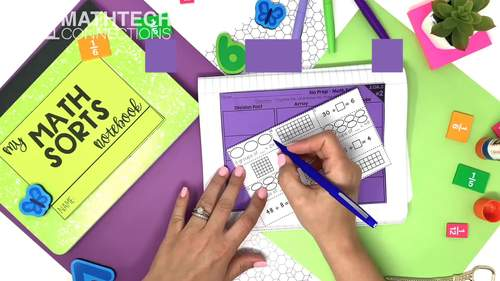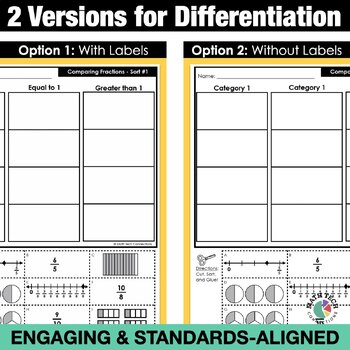EASEL BY TPT

Total:
\$0.00

# Math Interactive Notebook 3rd Grade Math Sorts Year Long Math Review Centers

Rated 4.88 out of 5, based on 1494 reviews
1.5k Ratings;
3rd
Subjects
Resource Type
Standards
Formats Included
• Zip
Pages
81 math sorts + notebook dividers + lesson plan template
\$20.00
List Price:
\$40.00
You Save:
\$20.00
Bundle
\$20.00
List Price:
\$40.00
You Save:
\$20.00
Bundle

#### What educators are saying

Amazing resource to use! I love that it has the answer key so my students can individually check their work!
I use these during our math centers and also for end of the year review in group work. The students find them very engaging! I appreciate the various levels so I can differentiate for my students!!

#### Products in this Bundle (5)

##### Also included in
1. This third grade math bundle includes 3 best-selling math bundles! Included: Math Tri-Folds, Math Sorts, and Math Morning Work!This is a MUST have resource for your 3rd grade classroom. The resources included are flexible and may be used for morning work, whole group practice, guided math practice,
Price \$64.00Original Price \$116.50Save \$52.50
2. This 3rd grade math bundle includes no-prep math sorting activities that can be used as partner math centers, math warm ups, or math exit tickets. This 3rd grade math practice bundle includes both PRINT and DIGITAL format. - The printable format includes 81 cut-and-past activities- The paperless for
Price \$30.38Original Price \$60.75Save \$30.37

### Description

Math Sorting activities are engaging and encourage critical thinking! Easily differentiate this interactive math activity by using the sorts with or without the labels.

Ways to use: math warm ups, small group lesson, independent math center, partner activity, math assessment, or homework!

What is Included?

- 81 sorts that review third grade math standards.

- Math Sorts Binder - Pages that list all math sorts, standard, topic, and small group lesson plan page for notes

- Blank PowerPoint Template - Just add text boxes to the template and create your own sorts!

- Student Notebook Dividers - Students keep their math sorts notebook organized with these dividers. Includes learning goals! The dividers are now editable.

- Student Directions for Day 1 and Day 2 Activities

** Try 5 FREE Math Sorts **

Third Grade Math Skills in this Bundle:

3.NBT Sort Topics:

• Rounding to the Nearest 10 & 100 (3.NBT.1)
• Multiples of 10 (3.NBT.3)

3.OA Sort Topics:

• Multiplication (arrays/equal groups) (3.OA.1)
• Division (arrays/equal groups) (3.OA.2)
• Multiplication & Division (3.OA.3)
• Unknown Numbers in Equations (3.OA.4)
• Properties of Multiplication (3.OA.5)
• Fact Families (3.OA.6)
• Multiplication & Division within 100 (3.OA.7)
• Number Patterns (3.OA.9)

3.NF Sort Topics:

• Partitioning Shapes (3.NF.1)
• Fractions on a Number Line (3.NF.2)
• Equivalent Fractions, Comparing Fractions, & Whole Numbers as Fractions (3.NF.3)

3.MD Sort Topics:

• Telling Time & Elapsed Time (3.MD.1)
• Mass & Volume (3.MD.2)
• Graphs (3.MD.3)
• Measuring Length (3.MD.4)
• Measuring Area (3.MD.5-6)
• Perimeter (3.MD.4)

3.G Sort Topics:

• Categorizing Shapes (3.G.1)
• Area as a Unit Fraction of a Whole (3.G.2)

MORE MATH SORTS

MATH TRI-FOLDS

Total Pages
81 math sorts + notebook dividers + lesson plan template
Included
Teaching Duration
N/A
Report this resource to TPT
Reported resources will be reviewed by our team. Report this resource to let us know if this resource violates TPT’s content guidelines.

### Standards

to see state-specific standards (only available in the US).
Tell and write time to the nearest minute and measure time intervals in minutes. Solve word problems involving addition and subtraction of time intervals in minutes, e.g., by representing the problem on a number line diagram.
Measure and estimate liquid volumes and masses of objects using standard units of grams (g), kilograms (kg), and liters (l). Add, subtract, multiply, or divide to solve one-step word problems involving masses or volumes that are given in the same units, e.g., by using drawings (such as a beaker with a measurement scale) to represent the problem.
Draw a scaled picture graph and a scaled bar graph to represent a data set with several categories. Solve one- and two-step “how many more” and “how many less” problems using information presented in scaled bar graphs. For example, draw a bar graph in which each square in the bar graph might represent 5 pets.
Generate measurement data by measuring lengths using rulers marked with halves and fourths of an inch. Show the data by making a line plot, where the horizontal scale is marked off in appropriate units-whole numbers, halves, or quarters.
Recognize area as an attribute of plane figures and understand concepts of area measurement.